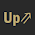# APFC Exam-2016 Solved Paper/Key

 Question Answer There are 20 girls and 30 boys in a class, and their respective average marks are found to be 55 and 58. The average marks of the entire class are 56.8 Consider an industry with the following features: Budgeted monthly fixed cost = Rs. 2, 20,000 Normal monthly output = 12000 per standard labour hour Standard variable overhead rate = Rs. 25 per labour hour What would be total factory overhead rate? Rs. 43.33 per labour hour There are four identically sized and shaped balls in a box, with only its top open. Each ball is of a different colour, these being: Green, Red, White and Blue, only one ball of each colour. Without looking into the box, one ball is randomly picked out and its colour is noted; then it is returned to the box. What are the chances that, in two successive draws, one may get the white ball and the red ball – in whichever order? 1/8 A cylindrical closed tank contains 36π cubic metres of water, and is filled to half of its capacity. When the cylindrical tank is placed upright on its circular base on level ground, the height of the water in the tank is 4 metres. But, when this tank is placed on its side on level ground, what will be the height of the surface of the water above the ground? 3 meters Data regarding inventory of a particular item of usage in the production activities of an organization are: the quantity in stock is 1500 units and the value of this stock is Rs. 1, 27, 500. (This works out to an average unit cost of Rs. 85) During the ensuing year X, an additional 300 units are purchased at a unit cost of Rs. 95. Consumption in production processes during the year X has been 600 units. Working by the First-in-First-out basis, the value of the residual inventory of the item at the end of the year X will be Rs. 1, 05, 000 In a race, the first four winners are to be awarded points. Each winner’s points must be 5 more than that of the next position winner. Total sum of the points to be awarded is 50. What will be the points for the third position winner? 10 Which of the following trends in FDI inflows are correct? In 2003-04, the FDI equity inflow percentage growth was negative. From 2004-05 to 2007-08, the FDI inflows were very high and positive. In 2008-09, the FDI inflows were positive, but had decreased relative to the previous year. In raising an object to given height by means of an inclined plane, as compared with raising the object vertically, there is a reduction in Force to be applied The mean and standard deviation of a set of 16 non- zero positive numbers in an observation are 26 and 3.5 respectively. The mean and standard deviation of another set of 24 non-zero positive numbers without changing the circumstances, are 29 and 3, respectively. The mean and standard deviation of their combined set of observations will respectively be 27.8 and 3.21 A sinusoidal transverse wave is travelling in string. Any point of the string moves in SHM with the same angular frequency as that of the wave
Next
Please report if you find any errors in the questions.
Keep watching the spaceThanks for reading APFC Exam-2016 Solved Paper/Key

←Previous
« Prev Post
Next→
Next Post »

1.Good effort . thank u

1.2.